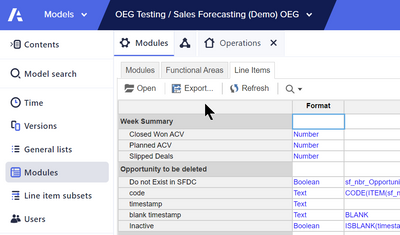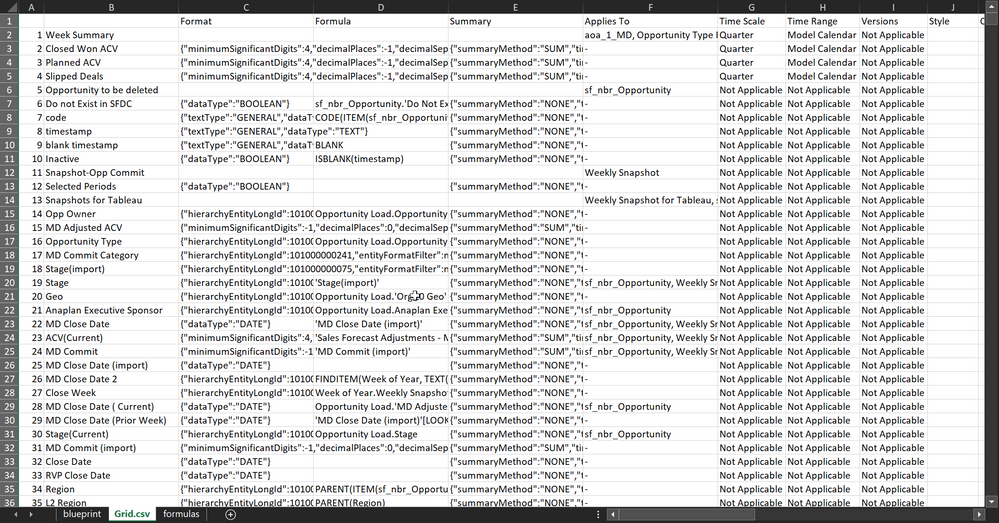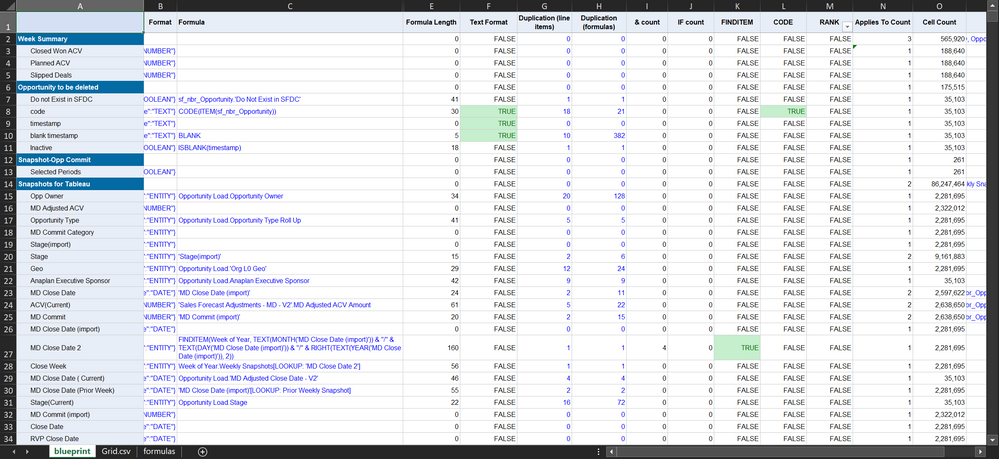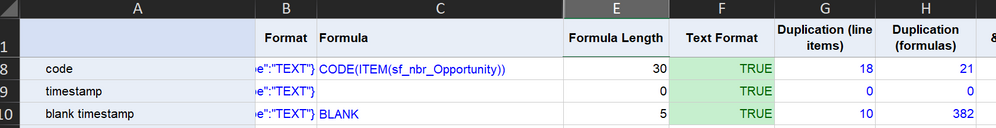# OEG Best Practice: Blueprint analysis: Model analysis techniques using Excel### What?

A model analysis technique using metadata from your Anaplan model imported in to Excel, utilizing purpose-built formulas to identify line items of interest

### Why?

We do this so that we have some extra information with which to arm ourselves when trying to optimize a model. It lets us look at every line item, apply the same rules to each, and highlight any line items that breach certain rules. We can then filter the worksheet and look at which line items we consider worth investigating first; for example, those we have highlighted as most likely to cause performance issues.

### How?

Begin by exporting line item "blueprint" from the third tab in Modules (found on the settings menu).
Export as CSV (Grid.csv)Import this in to Excel:Now we can begin to format the columns, hide those we are not interested in, and add columns for the analysis we want to accomplish.As an example, we've added a formula length column with the formula: =LEN(C2)
C2 is the formula column we have imported from the blueprint.

Here are the examples in the attached file:

 Formula Length `=LEN(C2)` Text Format `=ISNUMBER(FIND("TEXT",B2))` Duplication (line items) `=IF(C2=\$C\$2, 0, COUNTIF(C:C,C2))` Duplication (formulas) `=IF(C2=\$C\$2, 0, COUNTIF(C:C,"*"&C2&"*"))` & count `=LEN(C2)-LEN(SUBSTITUTE(C2,"&",""))` IF count `=(LEN(C2)-LEN(SUBSTITUTE(C2,"IF ","")))/LEN("IF ")` FINDITEM `=ISNUMBER(SEARCH("FINDITEM(",C2))` CODE `=ISNUMBER(SEARCH(“CODE(",C2))` RANK `=ISNUMBER(SEARCH(“RANK(",C2))` Applies To Count `=LEN(N2)-LEN(SUBSTITUTE(N2,",","")) + 1`

Some of the columns we've added speak for themselves; for example, formula length and text format.
The FINDITEM, CODE, etc. are all the same formula, but use different search terms. Note the use of an open parenthesis in the search term to look for the start of a function.
Duplication looks for that formula (row) in all the other rows, and shows how many times that exact formula is repeated in the model.
Duplication (formulas) is a similar search but uses a wild card to look for that text within the other rows. The example is looking for BLANK, and shows how many line items use BLANK in a formula.
The formula for '& count' shows how many instances of that character appear in the formula and will show any & in names as well. It may be better to search for " & " (with spaces).
The 'IF count' formula is similar to '& count', but we need to add /LEN("IF ") to account for more than one character in the substitute. Make sure the search term text is the same in both parts.

### ExampleIn this image, you can see the formula CODE(ITEM(sf_nbr_Opportunity)) is duplicated 18 times and then used a further 3 times in other line items (21 minus 18). This formula only applies to the list sf_nbr_Opportunity, so it should be put in to a list properties module (system module) dimensioned by only that dimension. Then this formula is calculated at the correct dimension in a module that other line items can reference when needed. The cell count of this line item is 35,103; so we're reducing the cells calculated for this from 631,854 (18 * 35103) to 35,103, a 94% reduction.
Do we need all those other line items now though?
Can they be removed and any references to those be changed to our new properties module?

#### Closing thoughts

I'm sure many of you are doing this already. If so then please share details on how you do it and what you look for.
Once you have a format, you'll find you can quickly apply this to new model blueprints. You could even drive the analysis page from a second sheet to make importing new blueprints easier.

You may even want to incorporate the results of a model open analysis file into this, looking up a line item and module name so that you can sort the blueprint by the performance data and match up the analysis timings to the formulas.

Please use this when trying to optimize performance. Even looking for duplicated formulas is a great start.

## Share your blueprint analysis formulas in the comments and we can grow this into something really useful!

Tagged:

•@MarkWarren Great article. For duplicates one thing I add is the summary as well in system modules. Occasionally, I need to create a Boolean filter that uses the same formula but has a different summary method, usually on time. For example, sometimes I want to see weeks and months, sometimes just weeks.

•Here's a new column to add; a count on the number of mappings in a SUM, where X[SUM:Y, SUM:Z] would return 2.

`=IFERROR((LEN(MID(C3,FIND("[SUM:",C3),FIND("]",C3,FIND("[SUM:",C3))-FIND("[SUM:",C3))) - LEN(SUBSTITUTE(MID(C3,FIND("[SUM:",C3),FIND("]",C3,FIND("[SUM:",C3))-FIND("[SUM:",C3)),"SUM:","")))/ LEN("SUM:"), 0)`

Look at that repetition, this formula needs optimizing! 😂
This only does the first SUM function in a formula.
Maybe someone can improve on this? Maybe it would need VBA?

•Hi @MarkWarren I tried the same last example you mentioned here with the CODE(ITEM(listName) I have 1 line item with +3M cells count so I created a new module with only 1 dimension (mylist) then I referenced that line item instead of using the formula but the Cell Count is exactly the same: +3M cells, I was expecting a cell count reduction but it didn't happen. Any ideas here? should I try something different?

•@JohanSmith  Does your target line item, the one referencing your code line item, need the other dimensions?
It may well do, if you are adding that code to another code for example.
You should only need to reference the original (the new one you created) if it is used in a formula or if you are using it in a saved view, say for an export.

The point of doing that change is both to reduce the cell count that formula is calculated over but also any repetitions of that formula; it's much cheaper to calculate once and refer to it many times.

Feel free to message me with screenshots if you need more help...